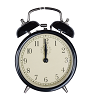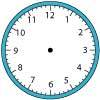You may also likeOn Time

On a clock the three hands - the second, minute and hour hands - are on the same axis. How often in a 24 hour day will the second hand be parallel to either of the two other hands?Right Time

At the time of writing the hour and minute hands of my clock are at right angles. How long will it be before they are at right angles again?Estimating Angles

How good are you at estimating angles?

Six Minutes Past Eight

Age 11 to 14 Short Challenge Level:

At 8 o'clock, the obtuse angle between the hands of the clock is 120°. In the following six minutes, the minute hand turns through an angle of 36° whilst the hour hand turns through an angle of 3° in the same direction (clockwise!). So the obtuse angle between the hands increases by 33°, to 153°.
This problem is taken from the UKMT Mathematical Challenges.
You can find more short problems, arranged by curriculum topic, in our short problems collection.